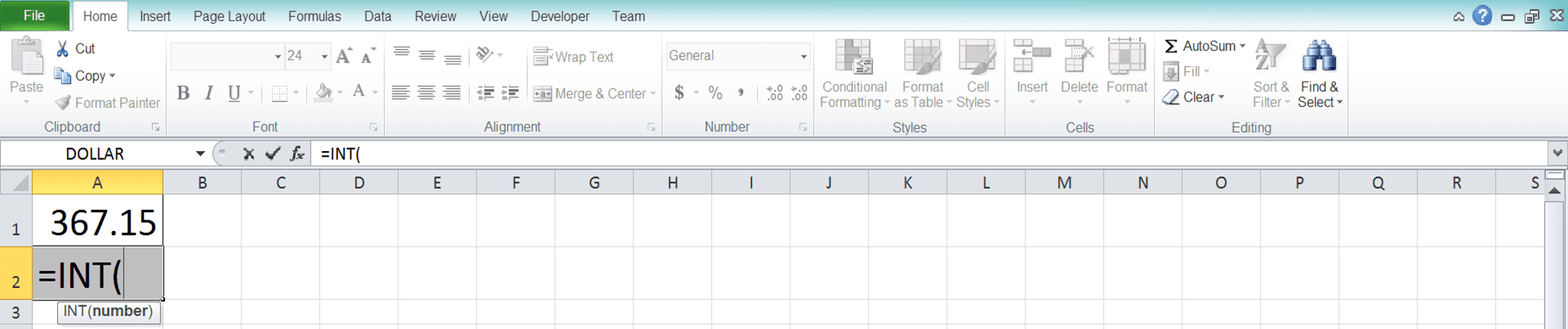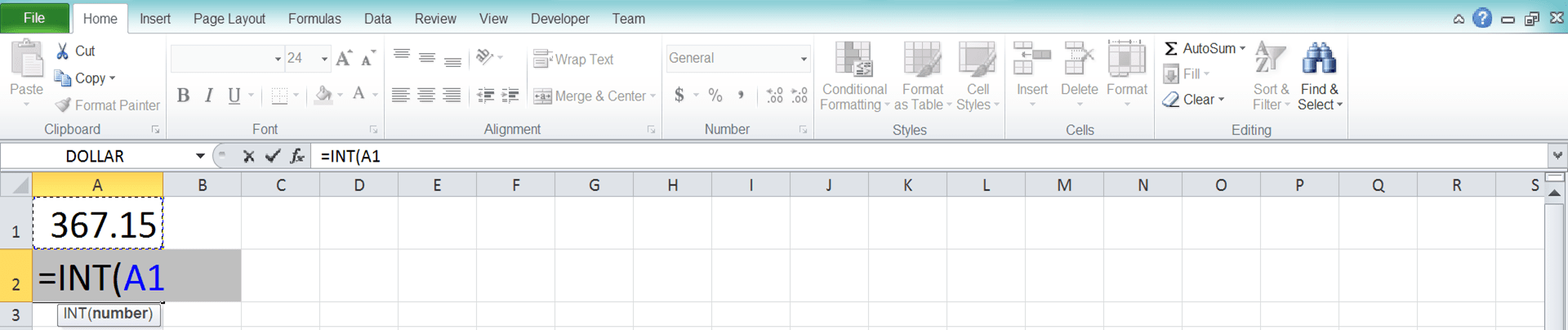How to Use the INT Formula in Excel: Functions, Examples, and Writing Steps - Compute Expert

# How to Use the INT Formula in Excel: Functions, Examples, and Writing Steps

Home >> Excel Tutorials from Compute Expert >> Excel Formulas List >> How to Use the INT Formula in Excel: Functions, Examples, and Writing Steps

In this tutorial, you will learn how to use the INT formula in excel completely.

When working with decimal numbers in excel, we sometimes need to round them down to their nearest integer. If you know how to use INT in excel, you can do that rounding process in a blink of an eye!

Want to know more about this INT formula and how to use it properly in excel? Read this tutorial until its last part!

Disclaimer: This post may contain affiliate links from which we earn commission from qualifying purchases/actions at no additional cost for you. Learn more

## What is the INT Formula in Excel?

The INT formula in excel is a formula that helps you to round down a decimal number into its nearest integer.

## INT Function in Excel

You can use INT to round down a number with decimal digits to its nearest integer.

## INT Result

The INT result is an integer that comes from the rounding down process of the decimal number you input into it.

## Excel Version from Which We Can Start Using INT

We can start using INT since excel 2003.

## The Way to Write It and Its Input

Here is the general writing form of INT in excel.

= INT ( number )

The only input you need to give to INT is the decimal number you want to round down with the formula.

## Example of Its Usage and Result

Here is an implementation example of INT in excel.You can see in the example how easy it is to use INT in excel. Just input the number you want to round down to its nearest integer and you are good to go!

If you input an integer to INT, then this formula won’t do anything to it. If you input a negative decimal number to INT, then it will round it away from zero.

## Writing Steps

After we discussed the INT writing form, input, and implementation example, now let’s discuss its writing steps. As INT only needs one simple input when you write it, its writing steps should be easy to understand.

1. Type an equal sign ( = ) in the cell where you want to put the INT result2. Type INT (can be with large and small letters) and an open bracket sign after =3. Input the decimal number you want to round down to its nearest integer or the cell coordinate where the number is4. Type a close bracket sign5. Press Enter
6. Done!## INT vs TRUNC

You may have known about the TRUNC formula before and notice that the formula is quite similar to INT. If you need to simply remove decimals from your number, then these two formulas are among the best ones to use.

But, what is the differences between these two formulas so you can choose the best one to utilize? Well, two differences stand out between them.

First of all, INT can only accept one input in its writing while TRUNC can optionally accept a second input. The optional second input of TRUNC is the number of decimals you want to see in its result. Thus, if you want to determine how many decimals you want to remove from your number, use TRUNC.

You can see the second difference if you want to remove decimals from a negative number. As INT rounds down a number to its nearest integer while TRUNC truncates decimals, their result will be different.

INT will give you a negative integer away from zero while TRUNC will give you a negative integer closer to zero. For a clearer understanding about this, you can see the illustration below.As you can see, INT and TRUNC give different results when we input negative numbers to them. INT rounds them down, away from zero, while TRUNC just truncates the decimals. Because of this, TRUNC makes them closer to zero in its results instead.

Choose whether to use INT or TRUNC based on the formula characteristics that suit your numbers processing needs best!

## Exercise

After you have learned how to use INT in excel, now let’s deepen your understanding by doing the following exercise.

### Instructions

Do the instructions by giving the answers on the gray-colored cell in each row!
1. Round the number down to its nearest integer!
2. Round down the division result of the number on the left with the number on the right to its nearest integer!
3. Round down the number on the left to its nearest integer. Then, subtract it with the number on the right!

If you input time data to INT, then you will get 00:00:00. That is because time data in excel is equivalent to a decimal number in the range of 0 to 1.

That means when you input time to INT, it will round it down to 0 in terms of number. That is equivalent to 00:00:00 in time data in excel.

Related tutorials you should read too:

Get updated excel info from Compute Expert by registering your email. It's free!